# 二、数据读取

``````# 读取csv数据
``````

# 三、数据概览

``````# 查看数据形状
df.shape
``````

``````# 查看前5行
``````

``````# 查看列信息
df.info()
``````

``````# 描述性统计分析
df.describe()
``````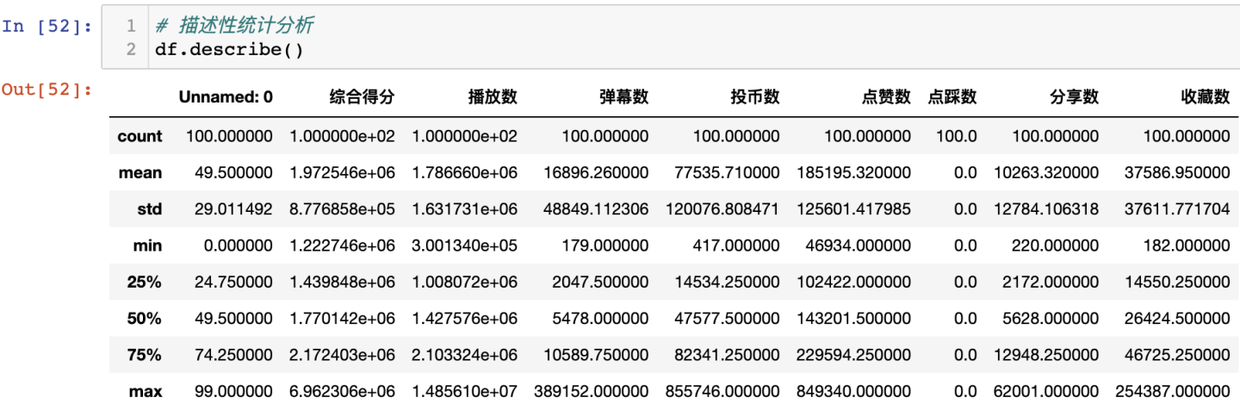# 四、数据清洗

``````# 查看空值
df.isna().any()
``````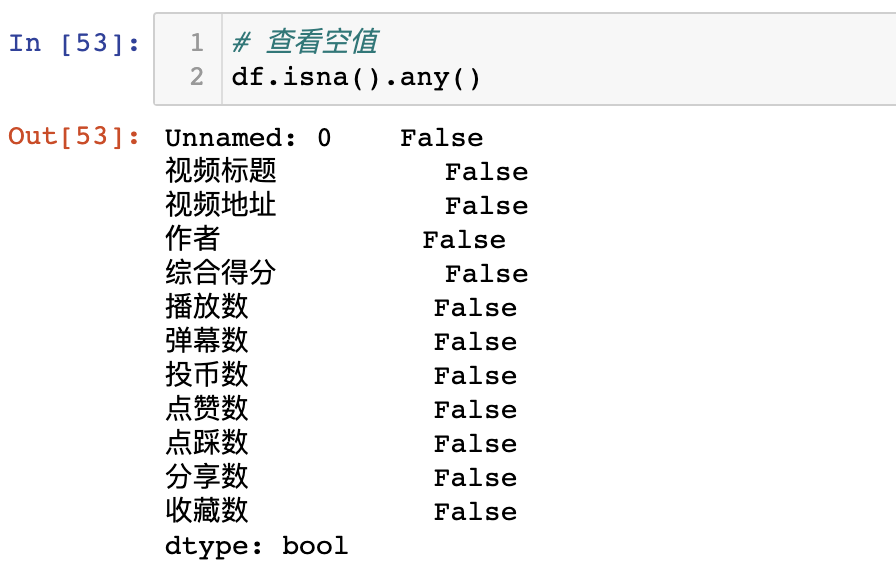每列都是False，没有空值。

``````#查看重复值
df.duplicated().any()
``````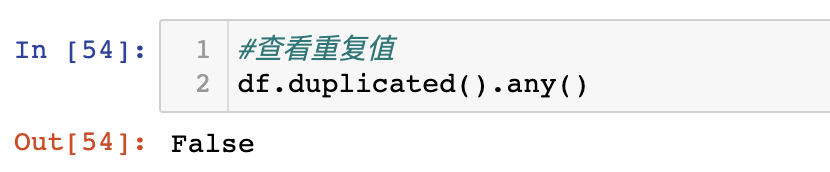False代表没有重复值。

``````# 删除没用的列
df.drop('点踩数', axis=1, inplace=True)
``````

# 五、可视化分析

## 5.2 饼图（Pie）

``````# 设置分段
bins = [1000000, 1500000,2000000, 2500000, 3000000, 10000000]
# 设置标签
labels = [
'100w-150w',
'150w-200w',
'200w-250w',
'250w-300w',
'300w-1000w'
]
# 按分段离散化数据
segments = pd.cut(score_list, bins, labels=labels)  # 按分段切割数据
counts = pd.value_counts(segments, sort=False).values.tolist()  # 统计个数
``````

## 5.3 箱形图（Boxplot）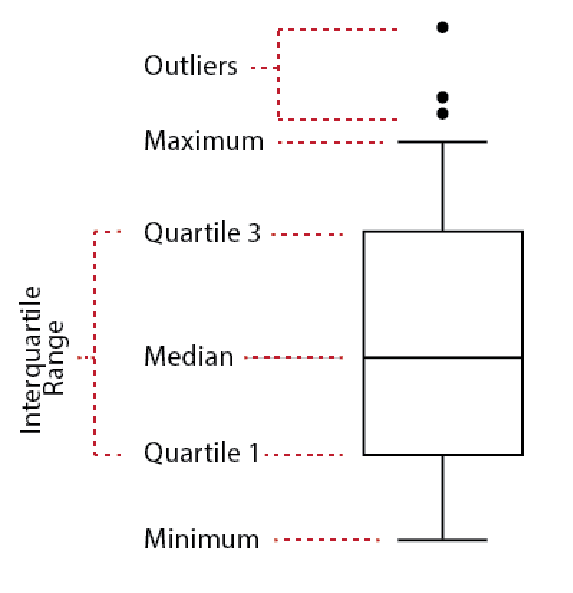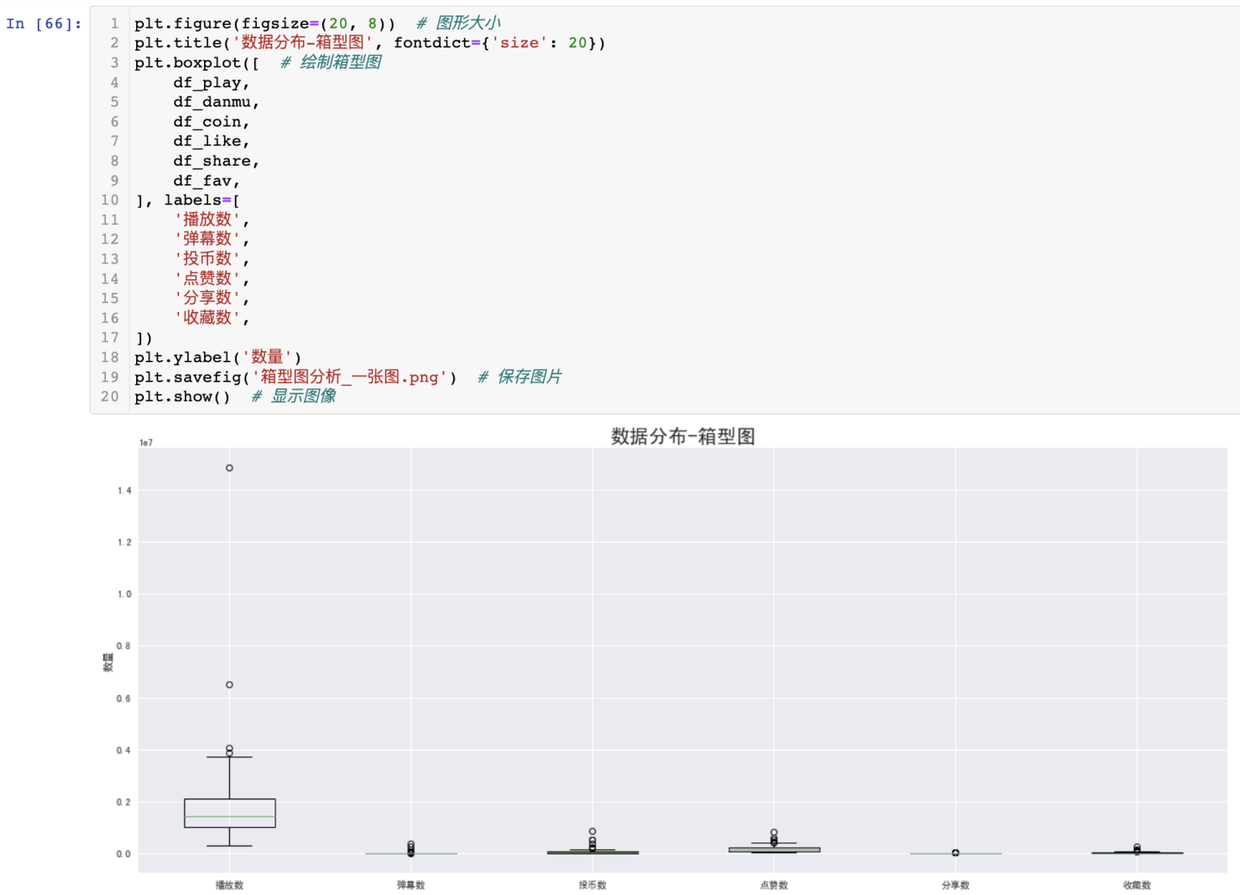## 5.4 词云图（wordcloud）2. 词云图和背景图的颜色分布，大体一致（color_func参数的作用）